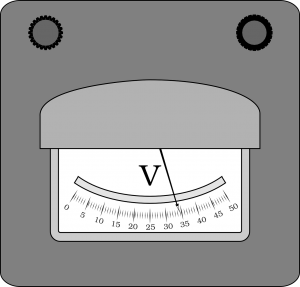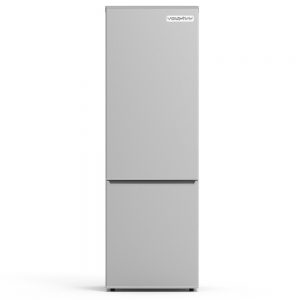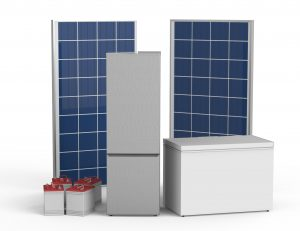# How to Spec a Solar System for Solar Fridges

### Where to start?

You’re deciding to use solar to go off-grid, great! The next question you may be asking yourself now is “What kind of solar system do I need?”. Have no fear, I will try my best to explain to you on how to calculate a system based on what your usage is.

Firstly, let’s get some terms out the way so we can have a better understanding as we proceed.

1. Load – Power consumption within a day of what you are using such as appliances, lights, and charging your phone or laptops. For our instance, we should use Amperage as it is easier to use than Watts.
2. Amperage – The strength of the electric current.
3. Voltage – The pressure electricity is moved. Usually it is supplied by a battery or generator.### Solar Fridges

Now for our situation let’s use our DC solar optimized refrigerator, the Voltray 11.1 CU. FT. The load/energy rating for this appliance is rated at 55.1 amps on a 24-hour consumption. Let’s factor in an extra 10 amps to this because the values are achieved by industry standard are done with doors closed settings. It’s because people are actually meant to use the appliance. It’s not art, it’s functional and mechanical, which is why we factor in extra amperage draw. Every time a door is opened, the cold air dumps out from any refrigerator, forcing the compressor to kick on and compensate for the energy loss. Which is why, the more you open a refrigerator, the more it has to run and the less efficient it becomes.

### The MathNow that we have the amperage which we calculated to be approximately 65.1 A. We can now move on to the math portion of this post. So, what kind of battery will we need? For the sake of this entry, we will be using a 12-volt golf cart battery system.

To calculate the wattage, we will use this formula: Amps = Wattage / Voltage. So, we have our Amperage and Voltage but we’re missing our Wattage. In order to get the wattage, which would be our amperage multiplied by our voltage, we get 65.1A x 12V so, it results in 781.2W.

If we factor that in most places around the world we would not have optimal daylight for more than 4 hours. So, let’s divide our total wattage of 781.2W by 4 hours which gives us a consumption rating of 195.3W/hr of real world usage (opening and closing doors). This means we would need a 195.3W minimal of wattage to recover our daily load.

Now that we have all our total numbers we know that we need a minimal of 195.3W. We usually like to round up to factor in unforeseen circumstances. We put these numbers to have 3 days of autonomy. This allows for downtime to repair a system if something comes up or if you have the nastiest weather such as rain for 3 days.

### Conclusions!

We would need to at least double the 65.1A rating. We don’t want our deep cycle batteries to drain any lower that 50% as this will damage the life cycle. This will need at least a 130.2Ahr battery system per day. In order to compensate for day to day usage, we usually scale our system for 3 days of autonomy. It’s just in case if you had a stormy/cloudy day or two. In order to scale up for three days, you can either take the daily system and increase it 3 fold, or take the consumption of the unit and increase it by a factor of 5-6. This is to factor in the 3 days of autonomy. This will give us roughly 325.5 – 390.6 Amp/Hour 12V battery system. This is the total amount of battery power we will require to run this system for 3 days without damaging the battery.To conclude you would need a solar panel or solar panels that will equal to 220ish Watts, 200 if you are really trying to save which I would not advise. Also, you would require a battery or equivalent to 325.5 – 390.6 Amp/hour 12V battery system.